Nonequispaced fast Fourier transform
Least squares
iNFFT

# Least squares

Let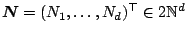. For given samples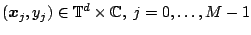, the index set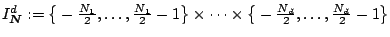, of frequencies, we construct a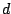-variate trigonometric polynomial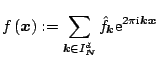such that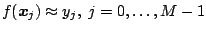. Turning this into matrix vector notation, we aim to solve the system of linear equations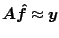(1)

for the unknown vector of Fourier coefficients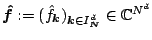. We denote the vector of the given sample values by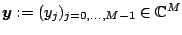and the nonequispaced Fourier matrix by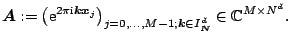For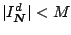, the linear system (1) is over-determined, so that in general the given data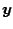will be only approximated up to a residual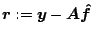. In order to compensate for clusters in the sampling set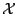, it is useful to incorporate weights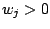and to consider the weighted approximation problem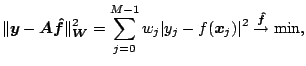(2)

where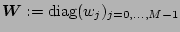. This library of C functions computes approximations of (2) with the CGNR method.
The algorithms are implemented by Stefan Kunis in ./solver. Related paper areKunis, S. and Potts, D.
Stability Results for Scattered Data Interpolation by Trigonometric Polynomials.
SIAM J. Sci. Comput. 29, 1403 - 1419,   (full paper ps, pdf)   2007